JEE  >  Test: Introduction To Complex Numbers

# Test: Introduction To Complex Numbers

Test Description

## 15 Questions MCQ Test | Test: Introduction To Complex Numbers

Test: Introduction To Complex Numbers for JEE 2023 is part of JEE preparation. The Test: Introduction To Complex Numbers questions and answers have been prepared according to the JEE exam syllabus.The Test: Introduction To Complex Numbers MCQs are made for JEE 2023 Exam. Find important definitions, questions, notes, meanings, examples, exercises, MCQs and online tests for Test: Introduction To Complex Numbers below.
Solutions of Test: Introduction To Complex Numbers questions in English are available as part of our course for JEE & Test: Introduction To Complex Numbers solutions in Hindi for JEE course. Download more important topics, notes, lectures and mock test series for JEE Exam by signing up for free. Attempt Test: Introduction To Complex Numbers | 15 questions in 15 minutes | Mock test for JEE preparation | Free important questions MCQ to study for JEE Exam | Download free PDF with solutions
 1 Crore+ students have signed up on EduRev. Have you?
Test: Introduction To Complex Numbers - Question 1

### Find the result in the form a + ib of (2-√-25) / (1+√-16)

Detailed Solution for Test: Introduction To Complex Numbers - Question 1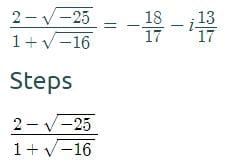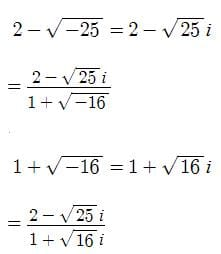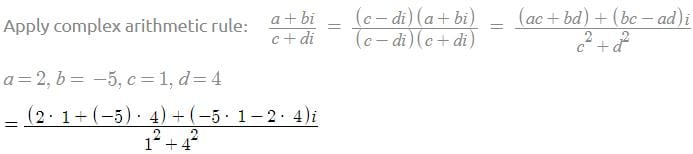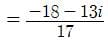Test: Introduction To Complex Numbers - Question 2

### Express the following in standard form :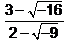Detailed Solution for Test: Introduction To Complex Numbers - Question 2

(3-4i) / (2-3i)*(2+3i) / (2+3i) = (6+9i-8i+12) / 13=(18/13)+(i/13)

Test: Introduction To Complex Numbers - Question 3

### Find the real numbers x and y such that : (x + iy)(3 + 2i) = 1 + i

Detailed Solution for Test: Introduction To Complex Numbers - Question 3

(x + iy)(3 + 2i) = (1 + i)
x + iy = (1 + i)/(3 + 2i)
x + iy = [(1 + i) * (3 - 2i)] / [(3 + 2i)*(3 - 2i)]
x + iy = (3 + 3i - 2i + 2) / [(3)2 + (2)2]
x + iy = (5 + i)/[ 9 + 4]
= (5 + i) / 13
=> 13x + 13iy = 5+i
13x = 5         13y = 1
x = 5/13         y = 1/13

Test: Introduction To Complex Numbers - Question 4

Express the following in standard form : (2 – √3i) (2 + √3i) + 2 – 4i

Detailed Solution for Test: Introduction To Complex Numbers - Question 4

Given: (2−√3i)(2+√3i) + 2 − 4i​

(2−√3i)(2+√3i) = 7

⇒ 7 + 2 - 4i

⇒ 9 - 4i

Test: Introduction To Complex Numbers - Question 5

Find the reciprocal (or multiplicative inverse) of -2 + 5i

Detailed Solution for Test: Introduction To Complex Numbers - Question 5

-2 + 5i
multiplicative inverse of -2 + 5i is
1/(-2+5i)
= 1/(-2+5i) * ((-2-5i)/(-2-5i))
= -2-5i/(-2)^2 -(5i)^2
= -2-5i/4-(-25)
= -2-5i/4+25
= -2-5i/29
= -2/29 -5i/29

Test: Introduction To Complex Numbers - Question 6

Find the real numbers x and y such that : (x + iy)(3+2i) = 1 + i

Detailed Solution for Test: Introduction To Complex Numbers - Question 6

(x + iy) (3 + 2i)
= 3x + 2xi + 3iy + 3i*y = 1+i
= 3x-2y + i(2x+3y) = 1+i
= 3x-2y-1 = 0 ; 2x + 3y -1 = 0
on equating real and imaginary parts on both sides
on solving two equations
x= 5/13 ; y = 1/13

Test: Introduction To Complex Numbers - Question 7

Write in the simplest form: (i)-997

Detailed Solution for Test: Introduction To Complex Numbers - Question 7

(i-997) = 1/(i997), 1/((i4)249) × i

Since (i4) = 1, (i4) / i = (i3)

= - i  (Since i2 = -1 , therefore, i3 = - i)

Test: Introduction To Complex Numbers - Question 8

Express the following in standard form : (8 - 4i) - (-2 - 3i) + (-10 + 3i)

Detailed Solution for Test: Introduction To Complex Numbers - Question 8

(8 - 4i) - (-2 - 3i) + (-10 + 3i)
=> 8 - 4i + 2 + 3i-10 + 3i
=> 8 + 2 - 10 -  4i + 3i + 3i  =>0 + 2i

Test: Introduction To Complex Numbers - Question 9

Express the following in standard form : (2-3i)2

Detailed Solution for Test: Introduction To Complex Numbers - Question 9

(2-3i)2 = 4 + 9 (i)2 - 2.2.3i
= 4 - 9 - 12i     since, i2 = -1
= - 5 - 12 i

Test: Introduction To Complex Numbers - Question 10

Find the multiplicative inverse of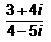Detailed Solution for Test: Introduction To Complex Numbers - Question 10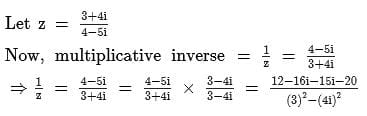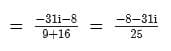Test: Introduction To Complex Numbers - Question 11

Express the following in standard form : i20 + (1 - 2i)3

Detailed Solution for Test: Introduction To Complex Numbers - Question 11

Given, i20 + (1 - 2i)3

We knoe that i = √-1

i2 = -1

Now put the values in given equation

= i20 + (1 - 2i)3

= ( i2)10 + { 1 - 8i3 - 6i + 12i}

= 1 +1 - 8i3 - 6i + 12i2

=1 +1 - 8i2.i1 - 6i + 12i2

=1 + 1 + 8i - 6i -12

=  -10 + 2i

Test: Introduction To Complex Numbers - Question 12

Imaginary part of −i(3i + 2) is:

Detailed Solution for Test: Introduction To Complex Numbers - Question 12

(-i)(3i) +2(-i) =-3(i^2)-2i =-3(-1)-2i =3-2i since i=√-1 =3+(-2)i comparing with a+bi,we get b=(-2)

Test: Introduction To Complex Numbers - Question 13

For a complex number a+ib, a-ib is called its:

Detailed Solution for Test: Introduction To Complex Numbers - Question 13

This is called conjugate of complex no.
z = a+ib. conjugate of z = a-ib
- sign is put before i

Test: Introduction To Complex Numbers - Question 14

Express the following in standard form :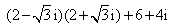Detailed Solution for Test: Introduction To Complex Numbers - Question 14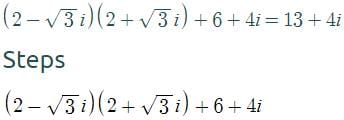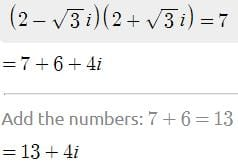Test: Introduction To Complex Numbers - Question 15

The multiplicative inverse of 3 – 4i is:

Detailed Solution for Test: Introduction To Complex Numbers - Question 15

Complete answers is in 3 steps:
1. Conjugate = 3+4i
2. Modulus = √3^2 + 4^2 =5
3. Multiplicative inverse = conjugate/square of modulus = 3+4i/5^2 = 3+4i/25

Information about Test: Introduction To Complex Numbers Page
In this test you can find the Exam questions for Test: Introduction To Complex Numbers solved & explained in the simplest way possible. Besides giving Questions and answers for Test: Introduction To Complex Numbers, EduRev gives you an ample number of Online tests for practice(Scan QR code)